GET VISIBLE! Advertise Here. Find Out MoreBedini - The Myth Of 'Free Electricity' By John Bedini From http://www.icehouse.net/john1/intro.html 5-27-16 This is also the basics for Tesla's energy shuttle circuits The two most important electrical energy statements ever made!!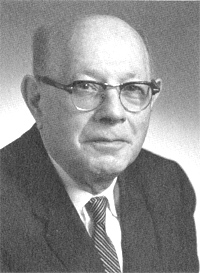Gabriel Kron 1901 to 1968 Kron, Gabriel. "...the missing concept of "open-paths" (the dual of "closed-paths") was discovered, in which currents could be made to flow in branches that lie between any set of two nodes. (Previously  following Maxwell  engineers tied all of their open-paths to a single datum-point, the 'ground').  That discovery of open-paths established a second rectangular transformation matrix... which created 'lamellar' currents..."  "A network with the simultaneous presence of both closed and open paths was the answer to the author's years-long search."  Gabriel Kron, "The Frustrating Search for a Geometrical Model of Electrodynamic Networks," Journal unk., issue unk., circa 1962, p. 111-128.  The quote is from p. 114. Kron, Gabriel. .  "When only positive and negative real numbers exist, it is customary to replace a positive resistance by an inductance and a negative resistance by a capacitor (since none or only a few negative resistances exist on practical network analyzers.)"  Gabriel Kron, "Numerical solution of ordinary and partial differential equations by means of equivalent circuits."  Journal of Applied Physics, Vol. 16, Mar. 1945a, p. 173.     EIGENVALUES AND EIGENFUNCTIONS Let it be assumed as an example that the V function is a potential well (Fig. 4a). Then in the network the corresponding positive resistances assume either a constant or a zero value as shown in Fig. 4b. In practice it is sufficient to extend the network to, say, twice the width of the potential well on either side. Let now a d.c.(or a.c.) generator be inserted anywhere in the network parallel with one of the negative resistances (inductors), as shown. If the values of all the negative resistances -E?x (capacitors) are simultaneously varied by the same amount, it will be found that the current (reactive current) in the generator varies and at some value of E?x becomes zero. It should be noted that while a current (reactive current) flows in the generator the circuit does not satisfy the differential equation, since at one point in the network (where the generator is connected) the currents do not add up to zero (as required by the equation) but to the generator current. That is, while a current flows in the generator, the voltages ? do not represent solutions of the differential equation. Hence, only those network conditions are of interest in which the generator current is zero.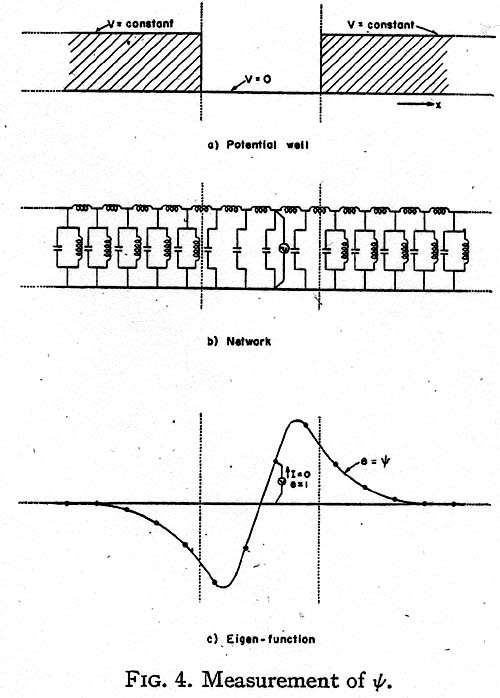Now a value E of the negative resistances, at which the generator current becomes zero, represents a state at which the circuit is self-supporting and has a continuous existence of its own without the presence of the generator, as the negative resistances just supply the energy consumed by the positive resistances. (If the circuit contains inductors and capacitors, the circuit is a resonant circuit and it oscillates at its basic frequency.) E is then an eigenvalue En, while the voltage distribution across the capacitors (Fig. 4c) gives the corresponding eigenfunction ?n. When the generator current is positive the circuit draws energy from the source, and when the current is negative the circuit pumps back energy into the source. At zero generator current the circuit neither gives nor takes energy, and theoretically the generator may be removed. All values of E at which the current crosses the axes and becomes zero are eigenvalues of the equation and the corresponding voltage distribution curves are eigenfunctions. When the energy level E overflows the well, the discrete spectrum of eigenvalues changes into a continuous spectrum and the generator current is zero at all greater values of E. When the energy E changes sign, the negative resistances become positive resistances and at no value of -E may the circuit be self-supporting (as it contains only positive resistances). That is, the equation has no negative eigenvalues.     THE STATISTICAL MEAN OF OPERATORS To bring the measurements at the different energy levels to the same base, it is necessary to normalize the measured ? values so that the new values of ? satisfy the equation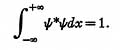The measured ? functions are normalized by plotting the square of ?. If the area under the curve is 1/N2, all values of ? are multiplied by N. Then N? is the normalized ?. (Actually N may contain an arbitrary phase ei? .) The statistical mean of an operator a for a state ? is defined as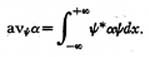Since (a?x)? is a current (reactive current) flowing through an admittance, ?*(a?x)? is the power (reactive power) in a single admittance. Hence the total power in a complete set of similar admittances, namely,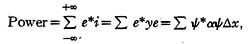represents the statistical mean of the corresponding energy operator. That is: 1. The total power in all the vertical negative resistors is the average value of E. (That is, E itself, since ? is an eigenfunction of H). 2. The total power in all the vertical resistors is the average value of the potential energy V. 3. The total power in all the horizontal resistors is the same as the total power in the vertical units, representing the average of the kinetic energy T = p2/ 2m. That is, the total power in all positive resistors is the same as the total power in all the negative resistors, or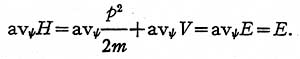THE THIRD MODEL Let the wave equation be divided by i?c, where ?c = v? = (E/ h), and multiplied by ?x: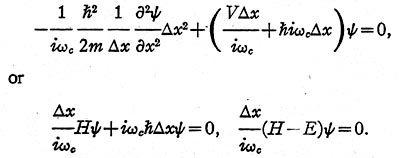In the present case: 1. The kinetic energy operator T is represented (Fig. 5) by a set of equal inductors in series, whose inductance L1 is (2m/ h2)?x.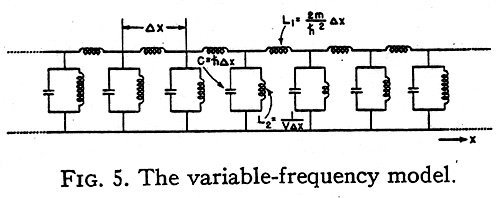2. The potential energy operator V is represented by a set of unequal coils in parallel, whose inductance L2 is 1/ V?x. 3. The total energy operator - E is represented by a set of equal capacitors in parallel whose capacitance is now h?x. (In the second model the capacitance was the unknown E?x.) The operand ? is again represented by voltages and the result of the operation a? by the same currents as in the first model. Their variation in time now is sinusoidal, with 2 p fc = ?c. Instead of varying the magnitude of the capacitors, now the frequency of the generator is varied, thereby varying the admittance of the capacitors, h?c = E (and those of the inductors). Again when the generator current becomes zero the circuit is oscillatory and self-supporting and the network represents a stationary solution of the equation. The corresponding eigenvalue is E = h? = h(?c )2, rather than h?c, because of the simultaneous variation of the reactance of the inductive coils. The eigenfunctions ? of the model and of the equation are, however, identical. As the currents in the horizontal inductors are (h2/2mi?c )??/ ?x, the results of an operation on ? by the momentum operator p = (h / i)?/ ?x are these currents divided by h2/ 2m?c. Hence, in the third model the momentum operator p may be represented by a set of equal horizontal coils with inductance L = ?x/h?c. One slight disadvantage of this third model is that as the energy E changes sign, the reactance of the capacitor j?ch cannot change signs. Since in most cases no eigenvalues exist in the negative energy range, this disadvantage is of little consequence. Of course, the second model with fixed frequency and variable capacitors works in all cases, since the capacitors simply become inductors when E changes sign. THE FREE PARTICLE IN ONE DIMENSION An interesting special case occurs when the potential V is zero everywhere. The one-dimensional equivalent circuit of such a free particle is a conventional transmission line extending to infinity in both directions (Fig. 6) in which the series inductance is 2m ?x/ h2 and the shunt capacitor is h?x.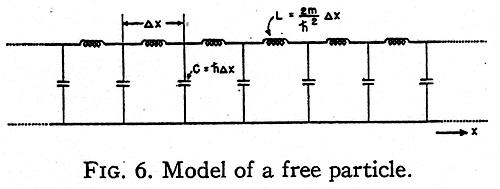It is well known that such a transmission line may maintain a standing wave at any frequency ?=?c between zero and infinity drawing no current from the generator. That is, the positive energy values form a continuous spectrum. If the transmission line is considered as the second type of model with variable capacitors, then at negative energy values E the capacitors also become inductors and the line cannot maintain a standing wave. The corresponding free particle also has no eigenvalue at the negative energy levels. MODELS ALONG CURVILINEAR AXES The three-dimensional Schrödinger equation for a single particle is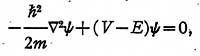where ?2 is the Laplacian operator in curvilinear coordinates. In order to establish a physical model for it, it is necessary to change it to a tensor density equation. (2) The above equation in orthogonal curvilinear coordinates may be changed to a tensor density form by multiplying it by h1h2h3 = vg givingIf the equation is multiplied through by ?u1?u2?u3, it represents the surface integral of grad ? around the six faces of a cube of space with volume h1h2h3?u1?u2?u3. The width ?ua may be arbitrary and different in the three directions. The corresponding equivalent circuit is shown in Fig. 7. For a free particle (V=0) it represents a generalization of the conventional one-dimen- sional transmission line to three dimensions.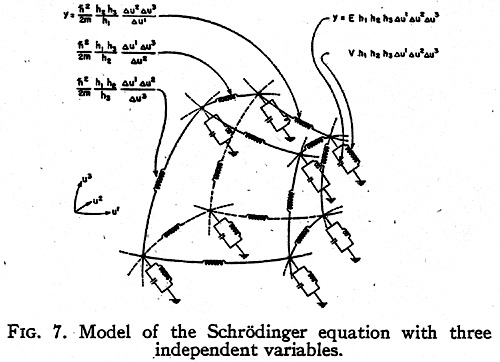It must be remembered that for a period during the 1960s Kron had refused to publish. Then he began to publish again, but very cautiously. Thus he was reluctant to take my advice about the new introduction. During two successive Saturdays of intensive discussion about this matter he told me that he was leaving his scientific colleagues behind and entering a new world, that hisnew discoveries would not be understood unless fully elaborated, and if elaborated would be plagiarized. In any event, he had no intention of tipping his hand until he had fully tested his new theories. As I read the new introduction, I find that he temporized. He did put his work in the perspective of its growth and development and did indicate the direction in which he was moving, but all in great generalities. These generalities may be informative, but if they are not then one would have to fall back on a comparison of the preliminary drafts of the new introduction - if such drafts are still extant. We know that with the successful development of "Diakoptics" Gabe turned his attention to new and greater challenges, to the comprehension of phenomena of a highly complex nature - multidimensional phenomena. From a philosophical point of view each such phenomenon would be considered complex because each was conceived as constituting a system not only of manifold operations, but of manifold heterogeneous operations. The prototype of this increasingly bold speculation and research was Gabes "crystal computer or vest pocket computer," as it was sometimes jokingly referred to, which utilized the simultaneous and heterogeneous activity of a crystal under stimulation of a single electric source. The important steps here seem to have been the perception of an analogy between the optical properties of a crystal under stimulation of a single source of light and the mathematical properties of an electromagnetic field surrounding an electric current, together with the successful use of topology to understand and manipulate them. I say that the "crystal computer" constituted a prototype because I believe this work was successfully accomplished in all its theoretical aspects long before his death, for Gabriel Kron showed me what he considered to be proof of the superiority of his new instrument. By this time, however, something else had entered his mind. I suspect that with the theoretical background of his work in developing the "crystal computer" it was not a big jump for the mind of a  Gabriel Kron to begin thinking of the universe itself as a crystal. I also suspect that what he was working on when he died was a general theory applicable to the universe as a whole system. After all the universe is a complex phenomenon in which many heterogeneous activities take place simultaneously. Perhaps Gabe had more than a vague intuition about short-cuts for interplanetary travel. But what was Gabriel Kron up too that he was very cautious about? I have been working in the energy field for over 30 years, I have come to the conclusion that there is no "Free Electricity". There is only a potential radiant charge that makes up a dipole.   This energy makes up everything we touch and use in our electrical circuits as we know them. The electrical circuits as we know them are flawed, meaning they burn up the dipole that is free in nature.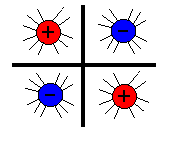If you kill the dipole you loose the energy. The dipole killer is the electron  current in the circuit. So therefore the term free electricity  only applies to those that have done  away with the current or have figured a way to block it from completing it's path through the circuit. Their are no meters to measure this radiant current, and when you catch it, it has the power of the universe and beyond. Good luck in your research . Their is only energy from the vacuum, known as radiant energy. to find out more about this you need to read Tom Bearden's book.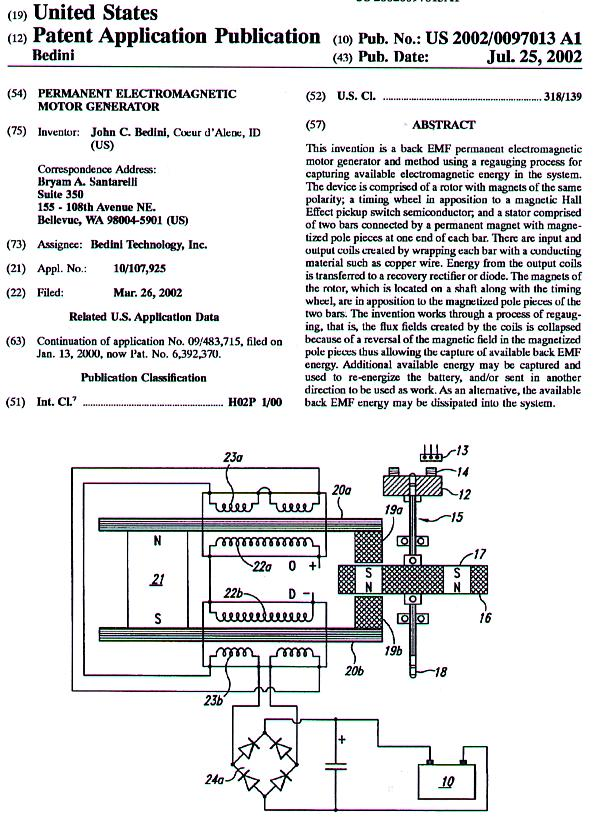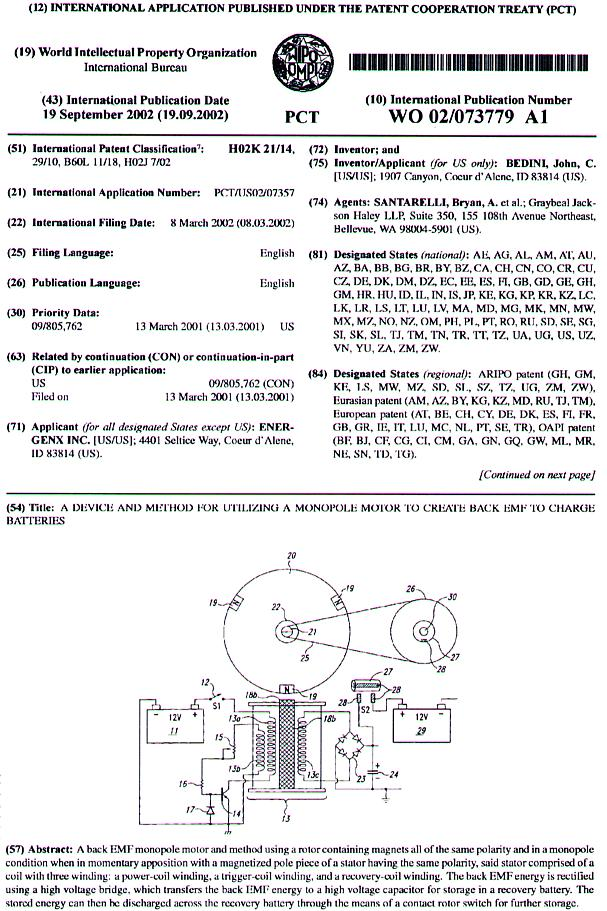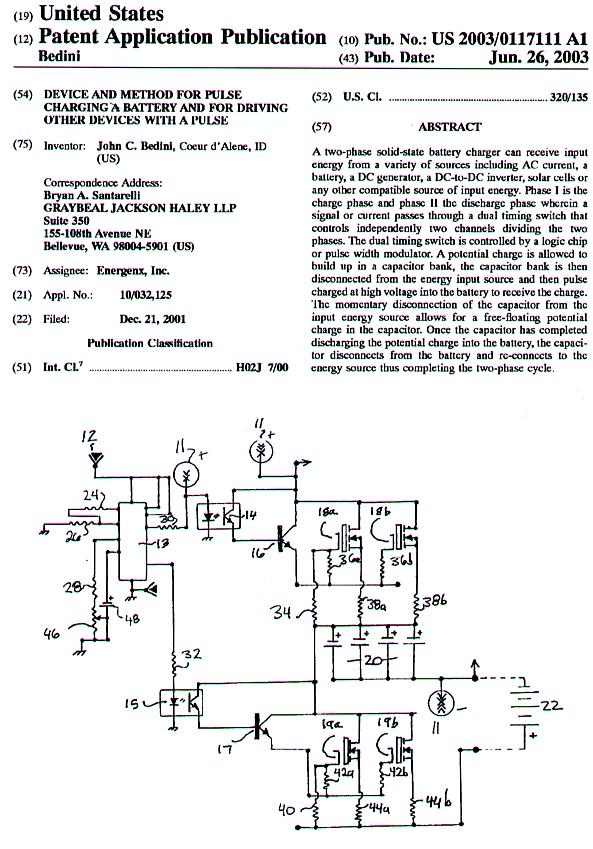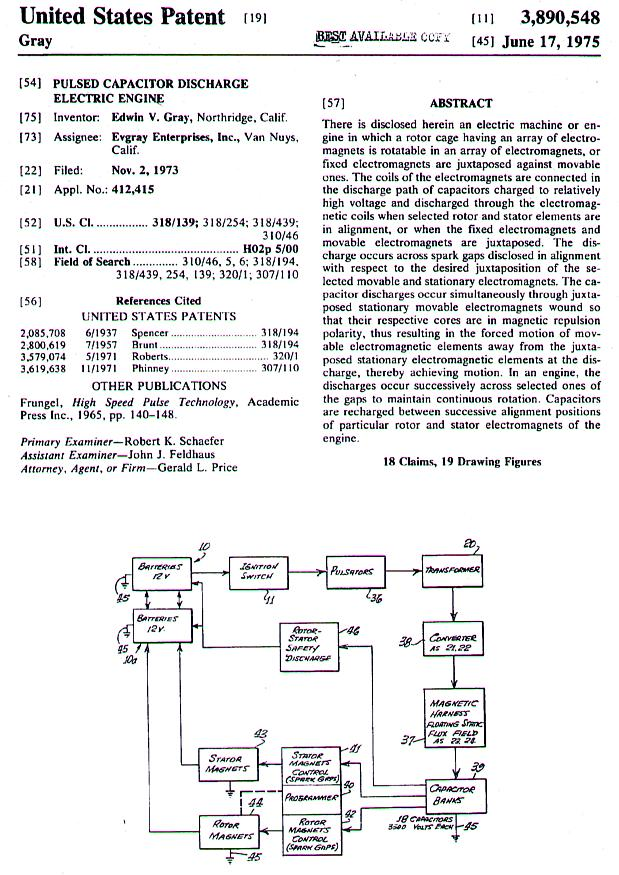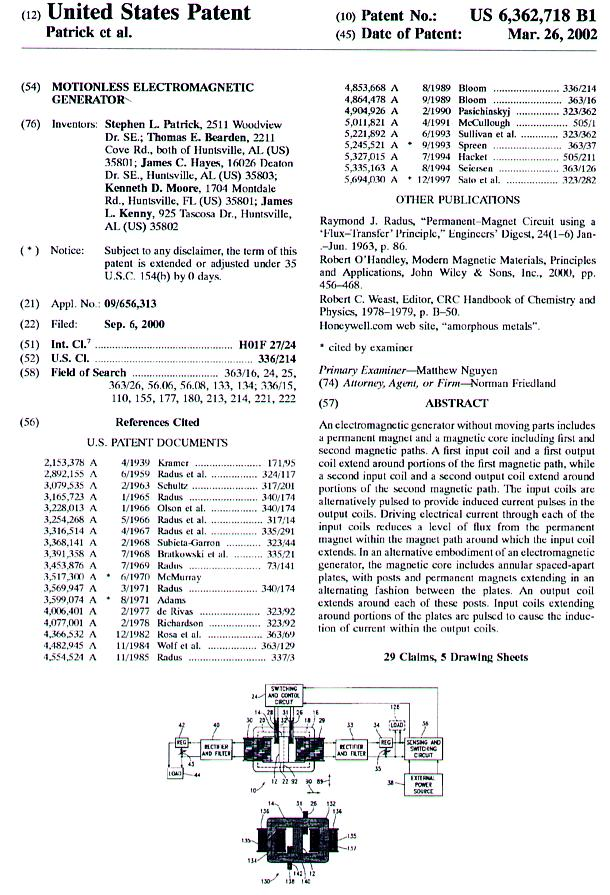These patents are the only key to unlocking this radiant energy.   Perhaps it is better in this present world of ours that a revolutionary idea or invention instead of being helped and patted be hampered and ill-treated in its adolescence- by want of means, by selfish interest, pedantery, stupidity and ignorance; that it be attacked and stifled ;  that it pass through bitter trials and tribulations, through the heartless strife of commercial existence. So all that was great in the past was ridiculed, condemned, combatted, suppressed - only to emerge all the more powerfully, all the more triumphantly from the struggle.    N. Tesla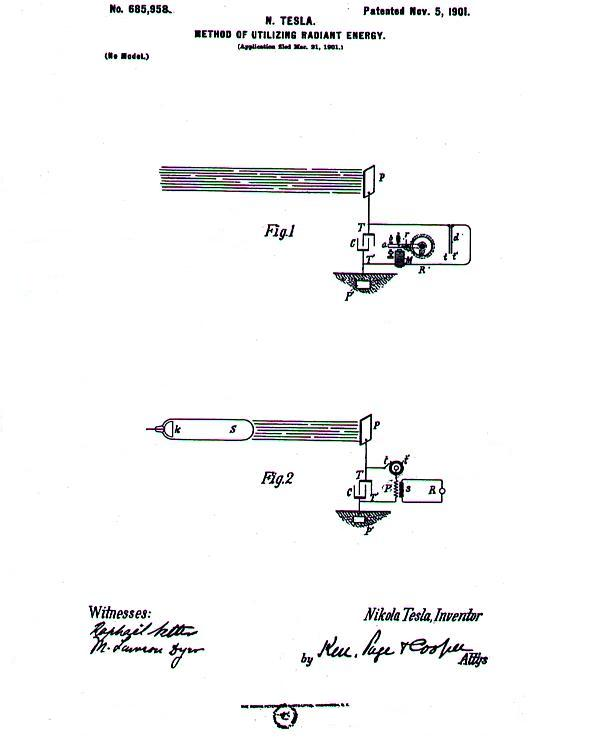"Today's scientist have substituted mathematics for experiments and they wander off through equation after equation and eventually build a structure which has no relation to reality." Nikola Tesla Here in the Tesla patent once again we see the use of radiant energy. As I have said above their is only a radiant current, and this current is passed through the system by the capacitor and only a capacitor. The next step in the system is the amount of time it takes to charge this capacitor, and then the amount of time it takes to discharge the capacitor for they all have their own time, and each one of these things must be in sync in their own time. It is so funny to sit here now and think that Benjamin Franklin had it all at his finger tips, if he would have used the capacitor the correct way. It's the most simple thing in nature to do, and the hardest for mankind to understand because of the mind blocks. Their is no math for radiant current, for are instruments do not measure it. It's even sick to see meters all over these machines, because their is no possible way to measure the Aether flow. The people have had it all along and yet a little "meter" has stood in their way from the truth. Meters only measure wasted energy in the system. Yes here it is, THE REAL McCoy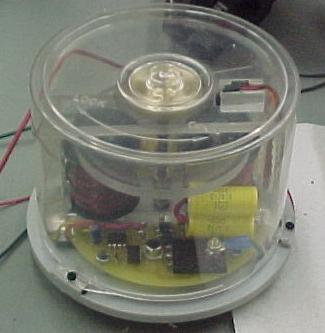(all patents are core technology, now using inverted radiant circuits) BTI systems inc.Radiant currents are just like Tesla said a gas in nature the transformation converter is the Capacitor.   An answer to David Thomson David this is great stuff and I admire your work, but the dipole we are talking about are two different things. first of all the math does not apply since their are no equations for it. The math of today's scientist only runs you around in equation after equation, "just like Tesla said". All are electrical equations are only designed to burn energy in current. I understand the The Casimir effect, but this does not have anything to do with the charge of a magnetic moment. this does not take any high voltage since the stress field is always there. Our circuits are designed to split the magnetic moment before it becomes magnetic, this is where the radiant comes from. If we go back to Tesla and what he was doing in the end we find out he was studying through small experiments how this Radiant gas behaved, and what shapes it worked best with. His work with different lattice was very detailed and this is where The Casimir effect comes into play. As for the Radiant work their only three patents by Tesla  which discusses anything, and no math. The only thing we have to use at this time is VQ without the I (current) but it should be Vr =Q ( voltage from the split Radiant = Charge) The only other factor is TIME to get the Vr. If a long time is required to collect charge in this split then C (capacitor) should be very small so that the highest V (voltage) is obtained. If the Vr is very quick in splitting this magnetic moment then C can be very big. Also frequency of this magnetic moment is very important. You must release the Radiant voltage into a radiant current de-void of any electrons. So what is charging the batteries in My system is pure Radiant Current. This means that since their is no electrons to pile up on the Ions of the battery nothing can move, this forces the Ions to change their state and they move on their own, this is nothing more then a impedance shift, if you have a low impedance in the battery you have real power, if you have high impedance in the battery you have nothing. Nature takes time to move at her own rate, so the battery may take a long time to charge but charge it will with a different form of energy. This brings me to a different point, That all that is driving the switching solid state wise is a difference in potential across the battery inverted. This then means that the whole flow theory is out the window, meaning that the semiconductors are working on potential charge de-void of electrons so their is no heat generated in the semiconductor. As for the battery it fill's in it's own electrons. I  must say that Tesla was right. John Answer Two David and Darren First of all with all due respect and admiration for your work, I must still state that My work has nothing to do with "Strong Charge", or Resonance in our circuits. My work is based on real working models, and these real working models are saying something much different than what the equations are saying. My magnetic moment is based on what is occurring in the front end circuit before the normal current builds up, and then to capture the radiant voltage and then split this voltage into a current de-void of electrons to charge the batteries.  So here we differ again I'm not looking for electrons to power anything, in your model you can not get away from the electrons. My models are telling me that the equations must be revised to work properly with My model. I not only have one model that is working I have 30 that do the same thing plus the solid state models. Radiant energy goes away when electrons enter the picture. Read very carefully what Tesla said about the power stations when they were DC powered, I think you will catch it. I have never seen anybody that did science right do the equations before they had a working model on the bench.  Also it's not "Professional" to speak of another fellow scientists working in the field as regurgitated Bearden concepts, when nobody has check the references to find out if he is right. I have worked with Tom Bearden for 30 +years and those theories come from my bench models that are working. Now others are getting to first base and soon they will be on third base, soon you will see these machines powering loads and charging their own batteries, then where will all the math models sit? If my patents were understood it would be found out that the Mono Pole motor is not a Motor, it is a mechanical Radiant oscillator with a one to one transformer inductively triggered. being a one to one transformer you can not get more out then you put in, unless something else is flowing through it, it does not fit the math models, so this washes the forward converter theory down the drain. I say this, if I take your model and understand it correctly, everybody should have their lights burning right now! What bench model do you have running on this theory?   I say these things to you with all do respect for your hard work. John Bedini These pages are for serious researchers only, doing serious work. I do not have time to answer questions on where you buy magnets or how you wind coils. You must be an engineering type of person and have machine experience. In the future I will be putting up pages on other systems according to Tom Bearden's new book. Energy From The Vacuum Concepts &Principles You may order this book from the Tom Bearden Website at www.cheniere.org/   I will be adding experiments, with the concepts of  Nathan Stubblefield's work on ground wave transmission. I have completed my test and have had very good results with over 10 miles  distance, even through the water pipes, rivers, streams in the local area. The circuits involved in these transmissions involve  vacuum tubes  and  high voltage. The researcher is warned that if  you have no experience in  ground wave radio transmission and FCC rules and regulations, stay away from these experiments. I take no responsibility for " your " actions, and you experiment at your own risk. If you cause interference to anything, you must stop your experiments, because the fine will be something you will never forget. By doing these experiments, you assume all responsibility. You will be shocked to see that you need no RF ( Radio Frequency) whatsoever. In the days of Nathan Stubblefield, they did not even understand the vacuum tube, so Nathan built many induction transformers to do this communication. If the experiments are understood , one may find that a whole new experimental field is now open. By phasing the transmission. it is possible to generate 3D special systems in sound and voice.   Quote from Stubblefield:  " I have solved the problem of telephoning without wires through the earth as Signor Marconi has of sending signals through space."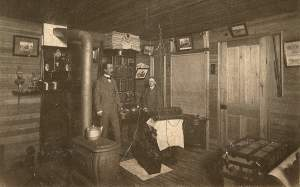Stubblefield in his workshop To get insight into Stubblefield go to www.nathanstubblefield.com and order the book

 Donate to Rense.com Support Free & Honest Journalism At Rense.com Subscribe To RenseRadio! Enormous Online Archives, MP3s, Streaming Audio Files,  Highest Quality Live Programs